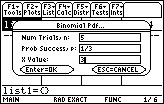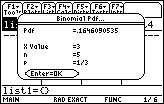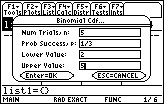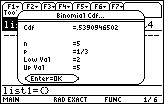# Knowledge Base

## Solution 13903: Calculating a Binomial Distribution on the TI-89 Family, TI-92 Plus and Voyage™ 200 Using the Statistics with List Editor Application.

### How can I calculate a binomial distribution on the TI-89 Titanium or Voyage 200 with the Statistics with List Editor Application?

The example below demonstrates how to calculate a binomial distribution on the TI-89 Titanium or Voyage 200.

Example: A hitter has a probability of 1/3 of getting a hit each time at bat, with each at-bat independent of other at-bat. In the next times at bat
Problem 1: What is the probability of getting exactly three hits?
Problem 2: What is the probability of getting at least two hits?

Solution 1: Computing the probability of getting exactly three hits.

• From the inside the Stats/List Editor App (Application), press [F5] to select Distr
• Select B:Binomial Pdf
• Enter the following:• Press [ENTER] twice to display the answer. The answer is pdf= .164609NOTE: This answer means the hitter has about 16% chance of getting three hits. If X value is left blank, the entire probability distribution will show in a list called “pdf.”

Solution 2: The probability of getting at least two hits is P(2)+P(3)+P(4)+P(5).

• From the inside the Stats/List Editor App (Application), press [F5]
• Select C:Binomial Cdf
• Enter the following:• Press [ENTER] twice and the answer .539095 should be displayed.Please see the TI-89 family, TI-92 Plus and Voyage 200 guidebooks for additional information.